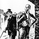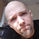# Strategy Code Example 2 - Time Limiting

จำนวนเข้าชม 10479
*** THIS IS JUST AN EXAMPLE OF STRATEGY TIME LIMITING CODE IMPLEMENTATION ***

This is a follow up to my example for risk management implementation here:

Code remains mostly unchanged, but now includes a time limiting implementation.

Relevant code blocks for the time factor are preceded with a comment stating:
• *** FOCUS OF EXAMPLE ***

Cheers!
```//@version=2

strategy(title = "Strategy Code Example", shorttitle = "Strategy Code Example", overlay = true, pyramiding = 0, default_qty_type = strategy.percent_of_equity, default_qty_value = 10, currency = currency.GBP)

// Revision:        1
// Author:          @JayRogers
//
// *** THIS IS JUST AN EXAMPLE OF STRATEGY TIME LIMITING ***
//
//  This is a follow up to my previous strategy example for risk management, extended to include a time limiting factor.

// === GENERAL INPUTS ===
// short ma
maFastSource   = input(defval = open, title = "Fast MA Source")
maFastLength   = input(defval = 14, title = "Fast MA Period", minval = 1)
// long ma
maSlowSource   = input(defval = open, title = "Slow MA Source")
maSlowLength   = input(defval = 21, title = "Slow MA Period", minval = 1)

// === STRATEGY RELATED INPUTS ===
// Risk management
inpTakeProfit   = input(defval = 1000, title = "Take Profit", minval = 0)
inpStopLoss     = input(defval = 200, title = "Stop Loss", minval = 0)
inpTrailStop    = input(defval = 200, title = "Trailing Stop Loss", minval = 0)
inpTrailOffset  = input(defval = 0, title = "Trailing Stop Loss Offset", minval = 0)
// *** FOCUS OF EXAMPLE ***
// Time limiting
// a toggle for enabling/disabling
useTimeLimit    = input(defval = true, title = "Use Start Time Limiter?")
// set up where we want to run from
startYear       = input(defval = 2016, title = "Start From Year", type = integer, minval = 0, step = 1)
startMonth      = input(defval = 05, title = "Start From Month", type = integer, minval = 0,step = 1)
startDay        = input(defval = 01, title = "Start From Day", type = integer, minval = 0,step = 1)
startHour       = input(defval = 00, title = "Start From Hour", type = integer, minval = 0,step = 1)
startMinute     = input(defval = 00, title = "Start From Minute", type = integer, minval = 0,step = 1)

// === RISK MANAGEMENT VALUE PREP ===
// if an input is less than 1, assuming not wanted so we assign 'na' value to disable it.
useTakeProfit   = inpTakeProfit  >= 1 ? inpTakeProfit  : na
useStopLoss     = inpStopLoss    >= 1 ? inpStopLoss    : na
useTrailStop    = inpTrailStop   >= 1 ? inpTrailStop   : na
useTrailOffset  = inpTrailOffset >= 1 ? inpTrailOffset : na

// *** FOCUS OF EXAMPLE ***
// === TIME LIMITER CHECKING FUNCTION ===
// using a multi line function to return true or false depending on our input selection
// multi line function logic must be indented.
startTimeOk() =>
// get our input time together
inputTime   = timestamp(syminfo.timezone, startYear, startMonth, startDay, startHour, startMinute)
// check the current time is greater than the input time and assign true or false
timeOk      = time > inputTime ? true : false
// last line is the return value, we want the strategy to execute if..
// ..we are using the limiter, and the time is ok -OR- we are not using the limiter
r = (useTimeLimit and timeOk) or not useTimeLimit

// === SERIES SETUP ===
/// a couple of ma's..
maFast = ema(maFastSource, maFastLength)
maSlow = ema(maSlowSource, maSlowLength)

// === PLOTTING ===
fast = plot(maFast, title = "Fast MA", color = green, linewidth = 2, style = line, transp = 50)
slow = plot(maSlow, title = "Slow MA", color = red, linewidth = 2, style = line, transp = 50)

// === LOGIC ===
// is fast ma above slow ma?
aboveBelow = maFast >= maSlow ? true : false
// are we inverting our trade direction?
tradeDirection = tradeInvert ? aboveBelow ? false : true : aboveBelow ? true : false

// *** FOCUS OF EXAMPLE ***
// wrap our strategy execution in an if statement which calls the time checking function to validate entry
// like the function logic, content to be included in the if statement must be indented.
if( startTimeOk() )
// === STRATEGY - LONG POSITION EXECUTION ===
strategy.entry( id = "Long", long = true, when = enterLong )
strategy.close( id = "Long", when = exitLong )

// === STRATEGY - SHORT POSITION EXECUTION ===
strategy.entry( id = "Short", long = false, when = enterShort )
strategy.close( id = "Short", when = exitShort )

// === STRATEGY RISK MANAGEMENT EXECUTION ===
strategy.exit("Exit Long", from_entry = "Long", profit = useTakeProfit, loss = useStopLoss, trail_points = useTrailStop, trail_offset = useTrailOffset)
strategy.exit("Exit Short", from_entry = "Short", profit = useTakeProfit, loss = useStopLoss, trail_points = useTrailStop, trail_offset = useTrailOffset)
```

## ความคิดเห็นWould it be possible to make a strategy only run during a certain time? Like between 9:00 to 10:00 for example.
ตอบกลับTheYangGuizi
Yes it should be entirely possible. Have a look at this script in the public library
ตอบกลับ